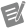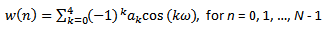# FFT Window Type Values

NI WLAN Analysis Toolkit 18.0 Help

Edition Date: October 2018

Part Number: 373724K-01

»View Product InfoDownload Help (Windows Only)

The following table lists the value descriptions for the NIWLANA_FFT_WINDOW_TYPE and NIWLANA_RECOMMENDED_SPECTRUM_FFT_WINDOW_TYPE attributes.

NIWLANA_VAL_WINDOW_UNIFORM (0)

No window is applied.

NIWLANA_VAL_WINDOW_HANNING (1)

Applies a Hanning window to the waveform using the following equation:

y[i] = 0.5 * x[i] * [1 - cos(w)]

 where w = (2)i/n n = waveform size

The Hanning window is useful for analyzing transients that are longer than the time duration of the window, as well as for general-purpose applications.

NIWLANA_VAL_WINDOW_HAMMING (2)

Applies a Hamming window to the waveform using the following equation:

y[i] = x[i] * [0.54 - 0.46cos(w)]

 where w = (2)i/n n = waveform sizeNote  Hanning and Hamming windows are similar. However, in the time domain, the Hamming window does not approach zero at the edge of the window as fast as does the Hanning window.
NIWLANA_VAL_WINDOW_BLACKMAN_HARRIS (3)

Applies a Blackman-Harris window according to the following equation:

y[i] = x[i] * [0.42323 - 0.49755cos(w) + 0.07922cos(2w)]

 where w = (2)i/n n = waveform size
NIWLANA_VAL_WINDOW_EXACT_BLACKMAN (4)

Applies an Exact Blackman window according to the following equation:

y[i] = x[i] * [a0 - a1cos(w) + a2cos(2w)]

 where w = (2)i/n n = waveform size a0 = 7938/18608 a1 = 9240/18608 a2 = 1430/18608

NIWLANA_VAL_WINDOW_BLACKMAN (5)

Applies a Blackman window according to the following equation:

y i = xi[0.42 - 0.50cos(w) + 0.08cos(2w)]

 where w = (2)i/n n = waveform size

A Blackman window is useful for analyzing transient signals, and provides similar windowing to Hanning and Hamming windows but adds one additional cosine term to reduce ripple.

NIWLANA_VAL_WINDOW_FLAT_TOP (6)

Applies a Flat Top window according to the following equation:

y[i] = x[i] * [a0 - a1cos(w) + a2cos(2w) - a3cos(3w) + a4cos(4w)]

 where w = (2)i/n n = waveform size a0 = 0.215578948 a1 = 0.41663158 a2 = 0.277263158 a3 = 0.083578947 a4 = 0.006947368

The fifth-order Flat Top window has the best amplitude accuracy of all the window types. The increased amplitude accuracy (±0.02 dB for signals exactly between integral cycles) is at the expense of frequency selectivity. The Flat Top window is most useful in accurately measuring the amplitude of single frequency components that have little nearby spectral energy in the signal.

NIWLANA_VAL_WINDOW_4_TERM_BLACKMAN_HARRIS (7)

Applies a 4-Term Blackman-Harris window according to the following equation:

y[i] = x[i] * [0.422323 - 0.49755cos(w) + 0.07922cos(2w)]

 where w = (2)i/n n = waveform size

The 4-term Blackman-Harris window has a side-lobe rejection in the upper 90 dB, with a moderately wide side lobe.

NIWLANA_VAL_WINDOW_7_TERM_BLACKMAN_HARRIS (8)

Applies a 7-Term Blackman-Harris window according to the following equation:

y[i] = x[i] * [a0 - a1cos(w) + a2cos(2w) - a3cos(3w) + a4cos(4w) - a5cos(5w) + a6cos(6w)]

 where w = (2)i/n n = waveform size a0 = 0.27105140069342 a1 = 0.43329793923448 a2 = 0.21812299954311 a3 = 0.06592544638803 a4 = 0.01081174209837 a5 = 0.00077658482522 a6 = 0.00001388721735.

The 7-term Blackman-Harris window has the highest dynamic range of the available windowing types and is ideal for signal-to-noise ratio applications.

NIWLANA_VAL_WINDOW_LOW_SIDE_LOBE (9)

The Low Side Lobe window further reduces the size of the main lobe. The following equation defines the Low Side Lobe window.where N = length of the window w = (2n/N) a0 = 0.323215218 a1 = 0.471492057 a1 = 0.17553428 a3 = 0.028497078 a4 = 0.001261367